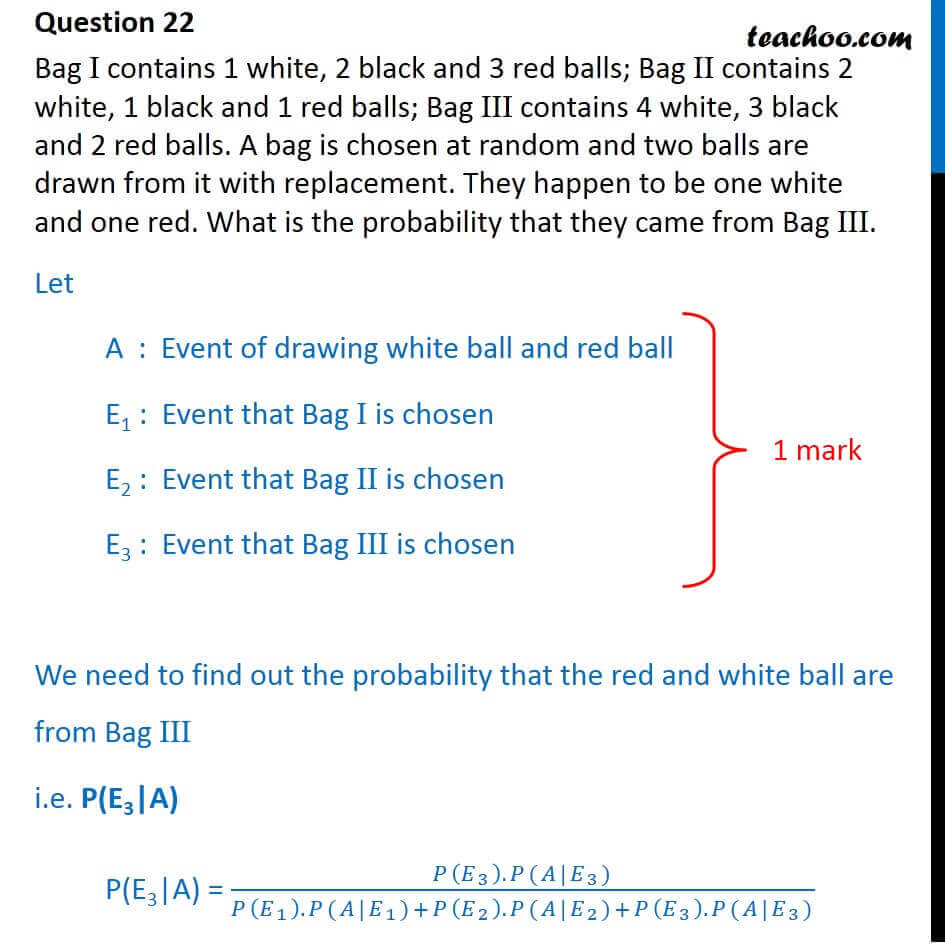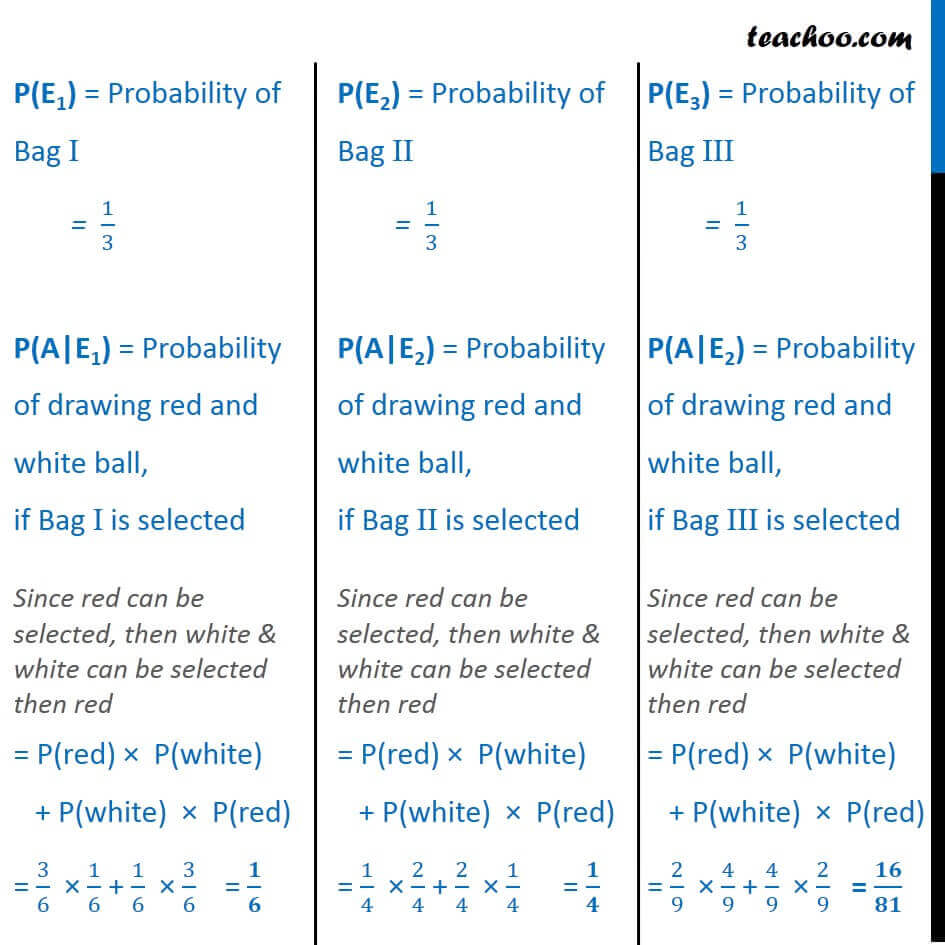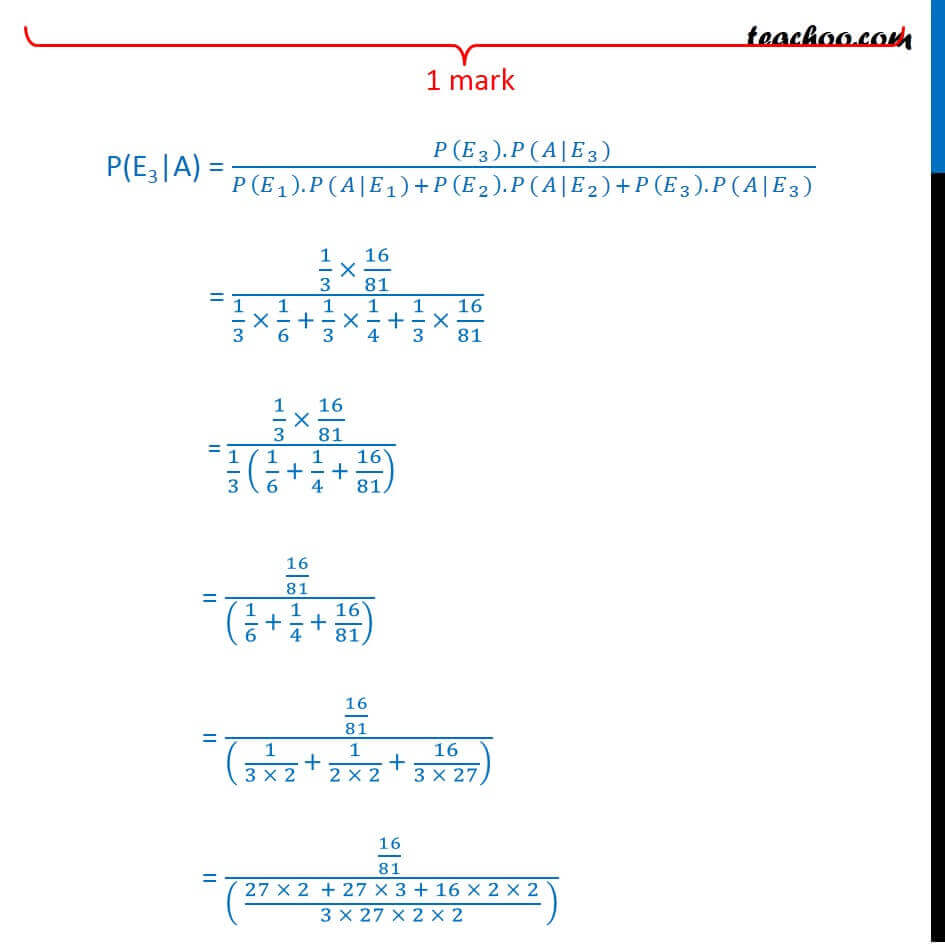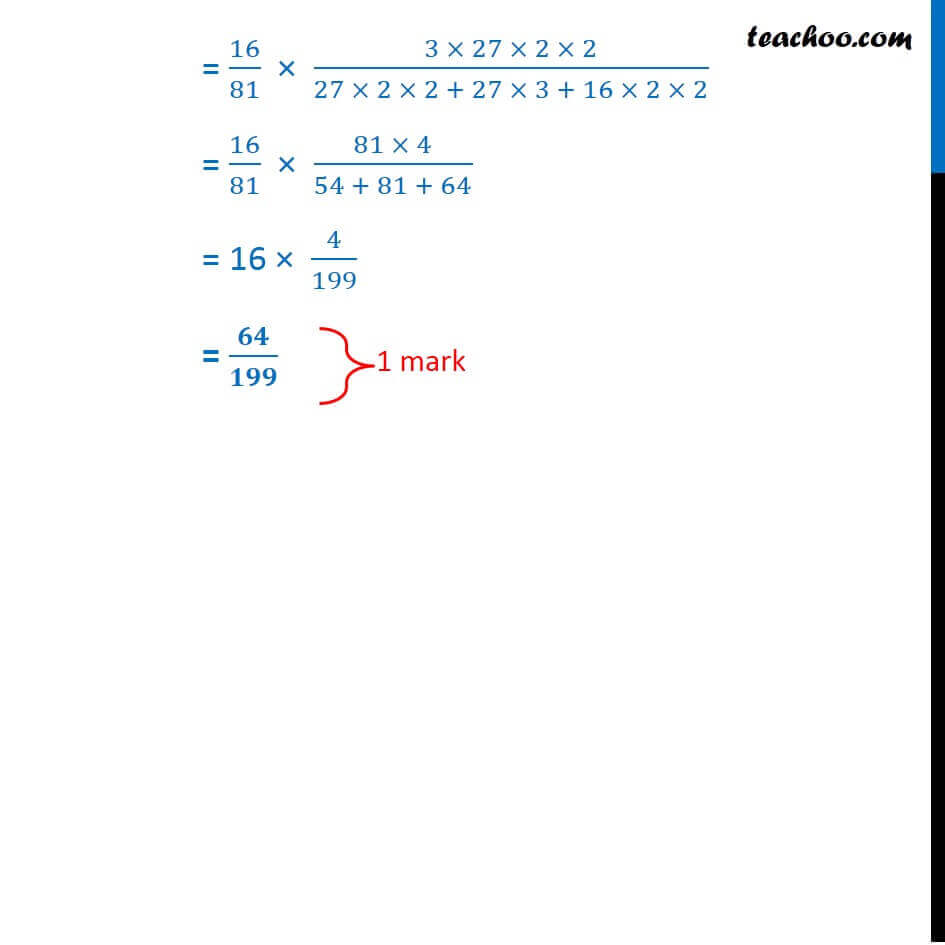CBSE Class 12 Sample Paper for 2018 Boards

Class 12
Solutions of Sample Papers and Past Year Papers - for Class 12 Boards

### Bag I contains 1 white, 2 black and 3 red balls; Bag II contains 2 white, 1 black and 1 red balls; Bag III contains 4 white, 3 black and 2 red balls. A bag is chosen at random and two balls are drawn from it with replacement. They happen to be one white and one red. What is the probability that they came from Bag III.

This is a question of CBSE Sample Paper - Class 12 - 2017/18.Learn in your speed, with individual attention - Teachoo Maths 1-on-1 Class

### Transcript

Question 22 Bag I contains 1 white, 2 black and 3 red balls; Bag II contains 2 white, 1 black and 1 red balls; Bag III contains 4 white, 3 black and 2 red balls. A bag is chosen at random and two balls are drawn from it with replacement. They happen to be one white and one red. What is the probability that they came from Bag III. Let A : Event of drawing white ball and red ball E1 : Event that Bag I is chosen E2 : Event that Bag II is chosen E3 : Event that Bag III is chosen We need to find out the probability that the red and white ball are from Bag III i.e. P(E3|A) P(E3|A) = (𝑃(𝐸_3 ).𝑃(𝐴|𝐸_3))/(𝑃(𝐸_1 ).𝑃(𝐴|𝐸_1)+𝑃(𝐸_2 ).𝑃(𝐴|𝐸_2)+𝑃(𝐸_3 ).𝑃(𝐴|𝐸_3)) "P(E1)" = Probability of Bag I = 1/3 P(A|E1) = Probability of drawing red and white ball, if Bag I is selected Since red can be selected, then white & white can be selected then red = P(red) × P(white) + P(white) × P(red) = 3/6 × 1/6 + 1/6 × 3/6 = 𝟏/𝟔 "P(E2)" = Probability of Bag II = 1/3 P(A|E2) = Probability of drawing red and white ball, if Bag II is selected Since red can be selected, then white & white can be selected then red = P(red) × P(white) + P(white) × P(red) = 1/4 × 2/4 + 2/4 × 1/4 = 𝟏/𝟒 "P(E3)" = Probability of Bag III = 1/3 P(A|E2) = Probability of drawing red and white ball, if Bag III is selected Since red can be selected, then white & white can be selected then red = P(red) × P(white) + P(white) × P(red) = 2/9 × 4/9 + 4/9 × 2/9 = 𝟏𝟔/𝟖𝟏 P(E3|A) = (𝑃(𝐸_3 ).𝑃(𝐴|𝐸_3))/(𝑃(𝐸_1 ).𝑃(𝐴|𝐸_1)+𝑃(𝐸_2 ).𝑃(𝐴|𝐸_2)+𝑃(𝐸_3 ).𝑃(𝐴|𝐸_3)) = (1/3 × 16/81 )/(1/3 × 1/6 + 1/3 × 1/4 + 1/3 × 16/81) = (1/3 × 16/81 )/(1/3 ( 1/6 + 1/4 + 16/81) ) = (16/81 )/(( 1/6 + 1/4 + 16/81) ) = (16/81 )/(( 1/(3 × 2) + 1/(2 × 2) + 16/(3 × 27)) ) = (16/81 )/(( (27 × 2 + 27 × 3 + 16 × 2 × 2)/(3 × 27 × 2 × 2) ) ) = 16/81 × (3 × 27 × 2 × 2)/(27 × 2 × 2 + 27 × 3 + 16 × 2 × 2) = 16/81 × (81 × 4)/(54 + 81 + 64) = 16 × 4/199 = 𝟔𝟒/𝟏𝟗𝟗